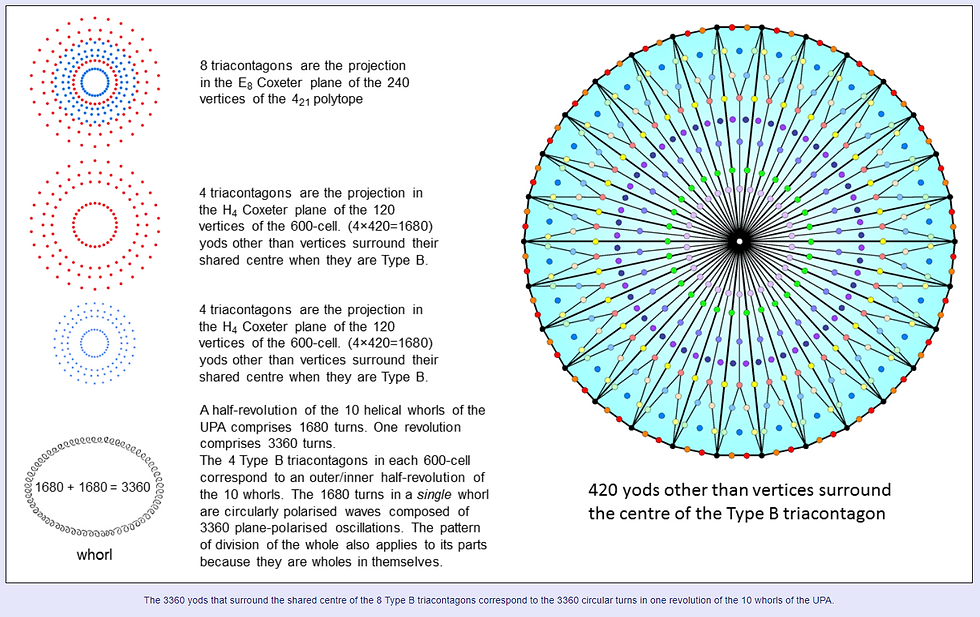Search

# tree of life superstring theory part 145

Updated: Nov 29, 2020The number of yods in a Type B n-gon = 15n + 1. It has 14n yods other than the (n+1) corners of its sectors. A Type B triacontagon (n=30) has 420 yods other than the 31 corners of its sectors. The H4 Coxeter projection of the 120 vertices of the 600-cell consists of 4 concentric triacontagons. (4×420=1680) yods surround their shared centre when they are Type B. This is the number of circular turns in one helical whorl of the UPA. The E8 Coxeter projection of the 240 vertices of the 421 polytope consists of 8 triacontagons (4 red, 4 blue) because the 4-dimension projection of these vertices are the 240 vertices of a compound of two 600-cells. Surrounding the centre of the 8 Type B triacontagons are 3360 yods. This is the number of turns in one revolution of the 10 whorls. Each set of 4 triacontagons that are its Petrie polygons corresponds to an outer or inner half-revolution of the 10 whorls. Alternatively, as each turn is a circularly polarised wave that is a supposition of two orthogonal plane waves oscillating 90° out of phase, there are 3360 plane waves in a whorl. The division:

3360 = 1680 + 1680

applies to both all 10 whorls and to a single whorl of the UPA. This is because the UPA is the subatomic Tree of Life and a whorl is one of its 10 Sephiroth, each of which must be regarded as a whole in itself, so that patterns characteristic of the holistic UPA must also apply to its holistic components. So the two half-revolutions of the 10 whorls embody the same number as the whorl does in terms of the two plane-wave components of each circularly polarised wave.